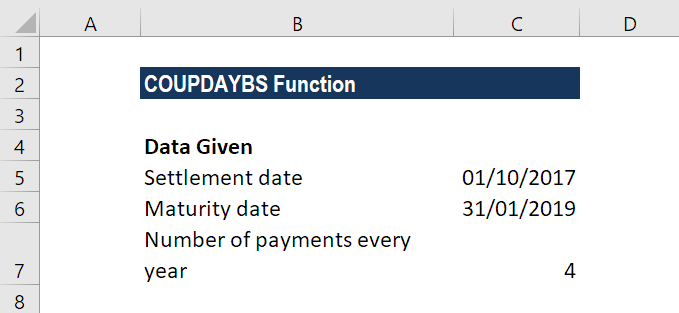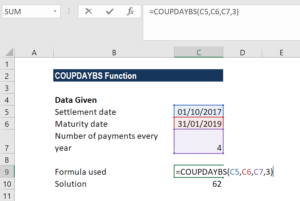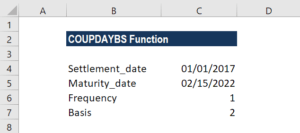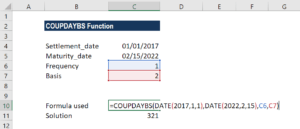# COUPDAYBS Function

Calculates the number of days from the start of a coupon’s period until the settlement date

## What is the COUPDAYBS Function?

The COUPDAYBS Function is categorized under financial functions. It helps calculate the number of days from the start of a coupon’s period until the settlement date. Coupon bonds are bonds that pay interest before maturity.

To know the number of days until the next payment, we can use the COUPDAYSBS function. Using the function, we get the number of days from the beginning of a coupon period until its settlement date.

### Formula

=COUPDAYBS(settlement, maturity, frequency, [basis])

The COUPDAYSBS function uses the following arguments:

1. Settlement (required argument) – This is the settlement date of a given security. It is the date after the security is traded to the buyer.
2. Maturity (required argument) – This is the date when the security expires.
3. Frequency (required argument) – This is the number of coupon payments per year. The argument can take a value of 1 (annual payment), 2 (semi-annual payments), or 4 (quarterly payments).
4. Basis (optional argument) – It specifies the day count basis to be used. It uses one of the following values:

BasisDay Count basis
0 or omittedUS(NASD) 30/360
1Actual/actual
2Actual/360
3Actual/365
4European 30/360

The function will default to zero when omitted. It indicates that the days in the month are counted using the US 30-day method with a 360-day year. When we enter 1 as the basis, the function uses the actual number of days in the month and year. Whereas, when we enter 2, it will count the actual days in the month with a 360-day year, while 3 will assume a 365-day year. When we enter 4 as the basis, it is the same as 1 except that it uses the European 30-day method.

### How to use the COUPDAYBS Function in Excel?

To understand the uses of the COUPDAYBS function, let’s consider a few examples:

#### Example 1

Let’s see how the function works when we are given the following data:Using the formula =COUPDAYBS(C5,C6,C7,3), we get the result 62 as the result. We used the Actual/365 as count days.#### Example 2

As the COUPDAYBS function doesn’t accept dates in text format, we need to convert them into DATE format. Let’s see an example to understand it. Suppose we are given the following data:As we need to find the number of days using the function, we need to first convert the dates given into text format.

The formula to be used would be =COUPDAYBS(DATE(2017,1,1),DATE(2022,2,15),1,2).The result we get here is 321. Excel first converted the dates into text format into proper dates and then calculated the number of days. Here, we used 1 as the frequency and 2 as the basis.

### Few notes about the COUPDAYBS Function

1. #NUM! error – Occurs in the following scenarios:
• When the settlement date is greater than or equal to (≥) the maturity date.
• When the frequency argument provided by the user is not equal to 1, 2 or 4.
• When the given basis argument is a number other than 0, 1, 2, 3 or 4.
2. #VALUE! error – Occurs in the following scenarios:
• When the given settlement date or the maturity date is not a valid date. Remember that we need to enter dates in date format or else use DATE function to convert them into proper dates. The function doesn’t work when the dates given are in text format. For example, =COUPDAYBS(“1/25/2023″,”11/15/2024”,2,1) = #VALUE
• Any of the arguments given is non-numeric.
3. The COUPDAYBS function truncates all arguments to Integers.

Thanks for reading CFI’s guide to the COUPDAYBS Excel function. By taking the time to learn and master Excel functions, you’ll significantly speed up your financial analysis. To learn more, check out these additional CFI resources:

• Excel Functions for Finance